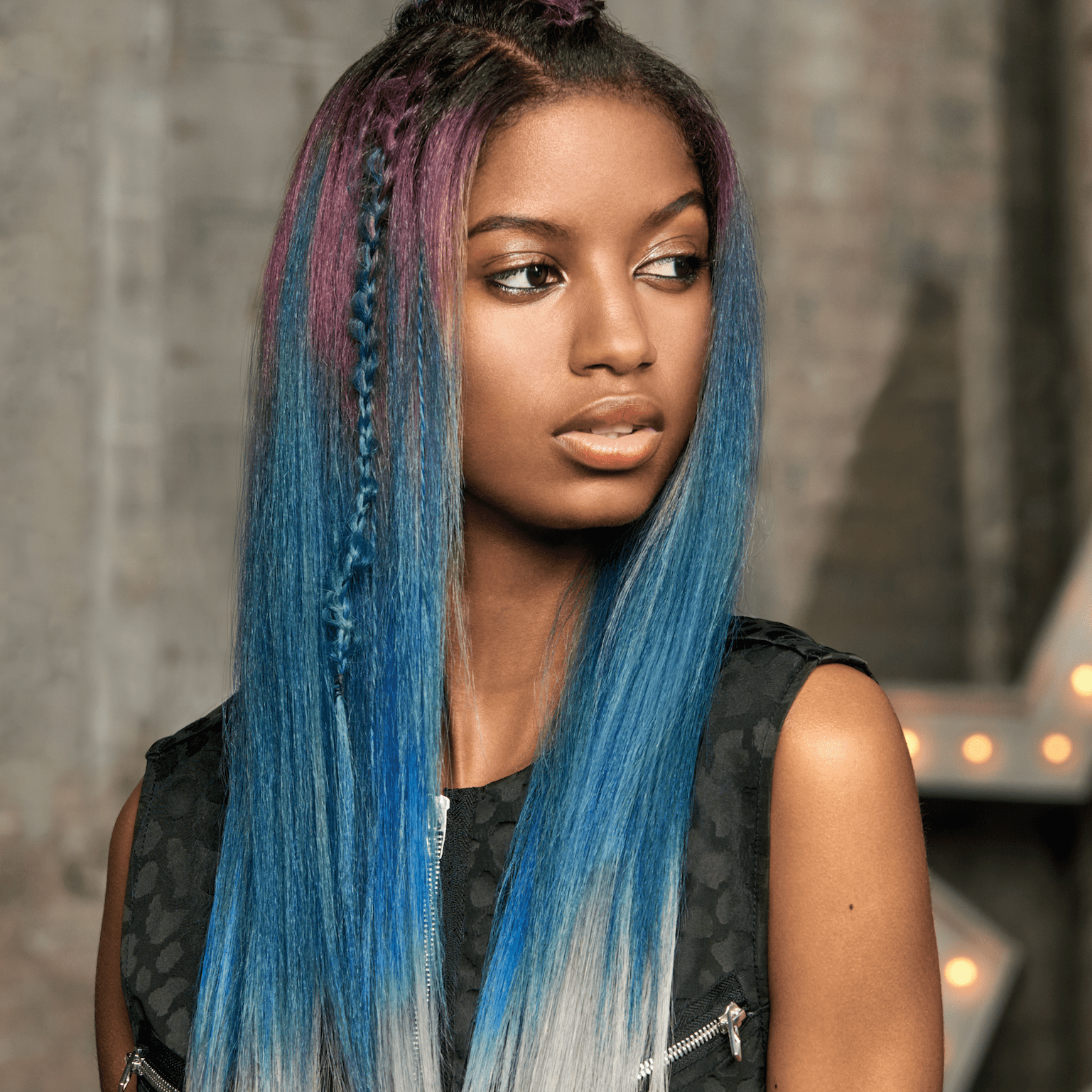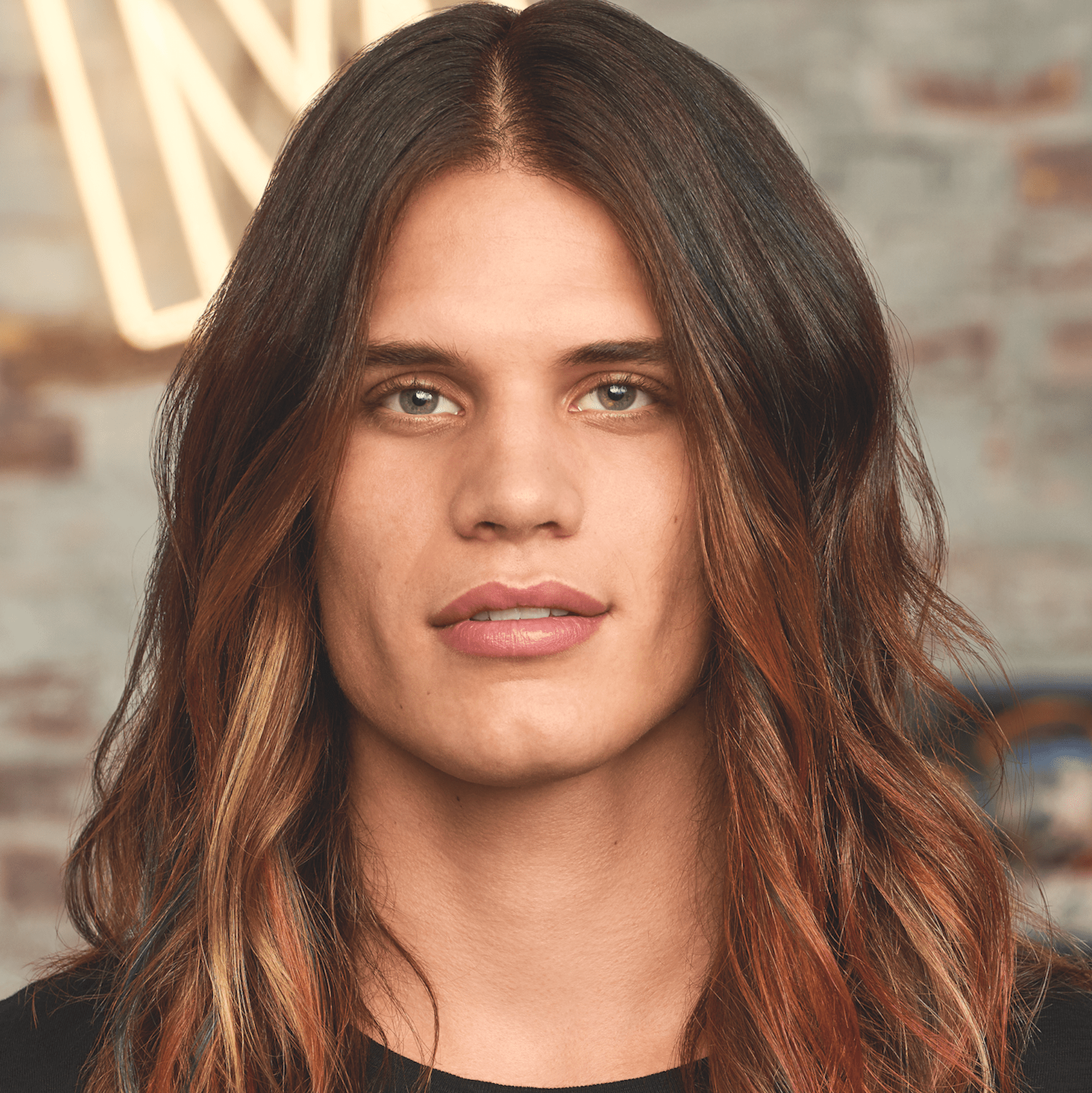Color Melting Hues
Last updated: June 01, 2017

# Color Melting Hues

ALL PHOTOS SLIDESHOWSEE THE COLLECTION# Color Melting Hues

Haircolor is constantly evolving—and right now the hottest new technique is color melting. So what do you get when you mix this cool new trend with soft fashion colors? A gorgeous opal color melt, and we have every detail you need to recreate it.

Colorist: Dan Csicsai, @dancsicsaihair; Danielle Keasling, @danielle.keasling; Dennis Rombout; Francis Bouchard; Mila Belova; Viktoriya Yeremchuk; Vitaly Pimenov
Manufacturer: Matrix, @matrix

## COLLECTION• Formula A

(3:1) Matrix Color Graphics Lacquer Blue + Clear

• Formula B

Color Graphics Lacquer Light Pink

• Formula C

(1:3) Color Graphics Lacquer Yellow + Clear

• Formula D

Equal Parts Color Graphics Lacquer Teal + Clear• Base Formula:

SC 8M

• Formula A:

Matrix CS SPV

• Formula B:

Matrix CS Berry Violet

• Formula C:

Matrix CS SPV + Quartz Pink 1:1

• Formula D:

Matrix CS Quartz Pink• Formula A:

Matrix 5VV

• Formula B:

Matrix Berry Violet

• Formula C:

Matrix Sapphire Blue

• Formula D:

Matrix Sapphire Blue + Clear 1:1

• Formula E:

Matrix 10P• Lightener:

Matrix Light Master 30vol

• Base:

Matrix SOCOLOR 5MV

• Formula A:

Matrix Light Indigo

• Formula B:

Matrix Light Pink

• Formula C:

Matrix Yellow